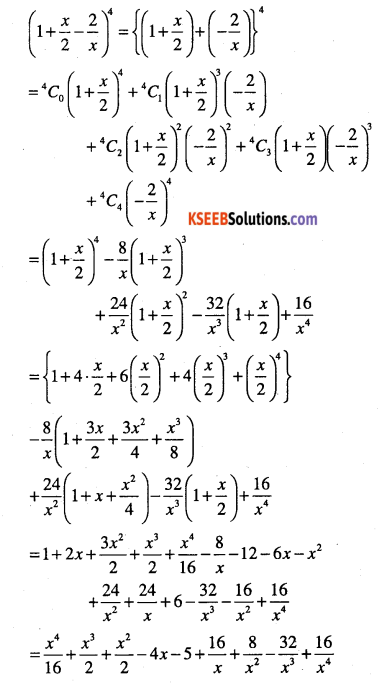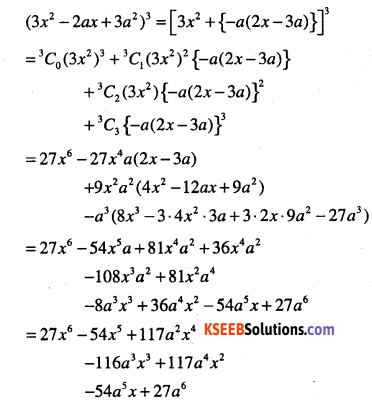# 1st PUC Maths Question Bank Chapter 8 Binomial Theorem

Students can Download Maths Chapter 8 Binomial Theorem Questions and Answers, Notes Pdf, 1st PUC Maths Question Bank with Answers helps you to revise the complete Karnataka State Board Syllabus and score more marks in your examinations.

## Karnataka 1st PUC Maths Question Bank Chapter 8 Binomial Theorem

Question 1.
State and prove Binomial theorem.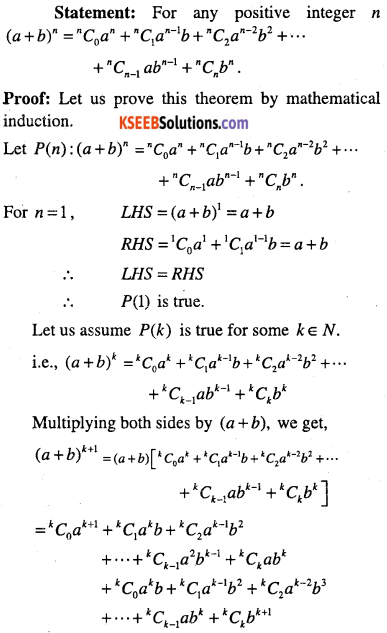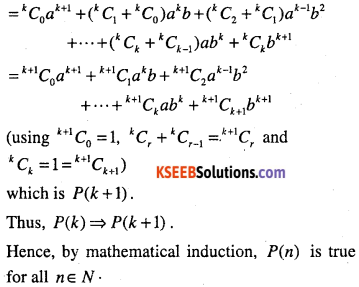Some observations in a binomial theorem:
(1) The expansion of {a + b)n has (n + 1) terms
(2) The coefficients nCr occurring in the binomial theorem are known as binomial coefficients.
(3) The indices of V go on decreasing and that of ‘a’ go on increasing by 1 at each stage.
i.e., for each term: index of a + index of b-n.
(4) Since nCr=nCn_r we have
nC0 =nCn, nCx =nCn_r and so on.
Thus the coefficients of the terms equidistant from the beginning and the end in a binomial theorem are equal.
(5) General term in (a + b)n: Tr+1 – nCr anrbr
(6) Middle terms in (a + b)n

• When ‘n’ is even, the middle term
$$=\left(\frac{n}{2}+1\right)^{t h} \text { term }$$
• When ‘n’ is odd ,the middle term are
$$\frac{1}{2}(n+1)^{n} \text { term an } \frac{1}{2}(n+3)^{n} \text { term }$$

(7) Taking a = x and b = -y in the expansion, we get (x-y)n =[x + (-y)]n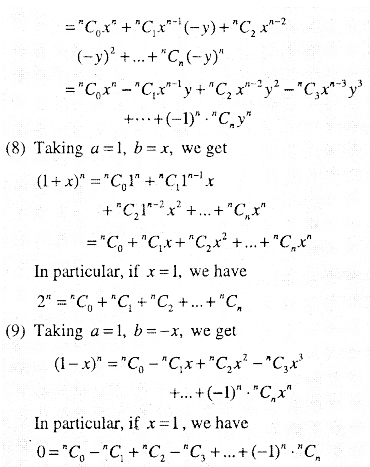Question 2.
Expand each of the expression
(i)$$\left(x^{2}+\frac{3}{x}\right)^{4}$$
(ii)$$(1-2 x)^{5}$$
(iii)$$\left(\frac{2}{x}-\frac{x}{2}\right)^{5}$$
(iv)$$(2 x-3)^{6}$$
(v)$$\left(\frac{x}{3}+\frac{1}{x}\right)^{5}$$
(vi)$$\left(x+\frac{1}{x}\right)^{6}$$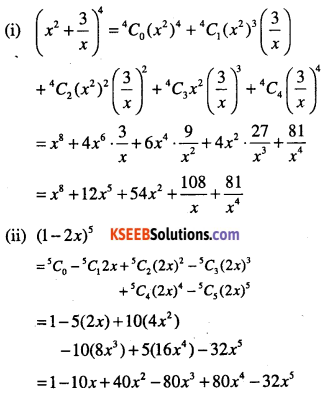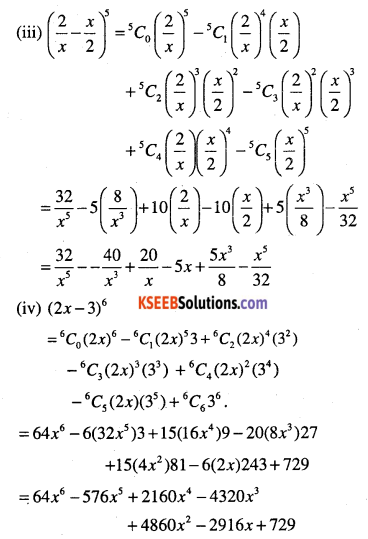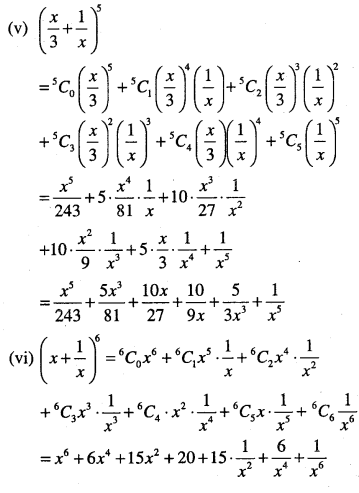Question 3.
Using binomial theorem, evaluate each of the following:
(i) (98)5
(ii) (96)3
(iii) (102)5
(iv) (101)4
(v) (99)5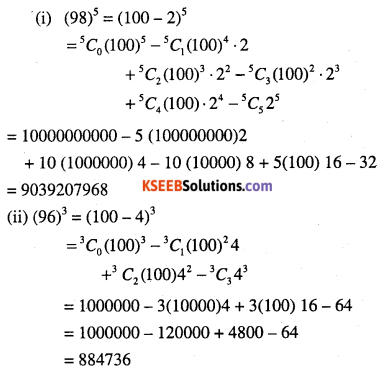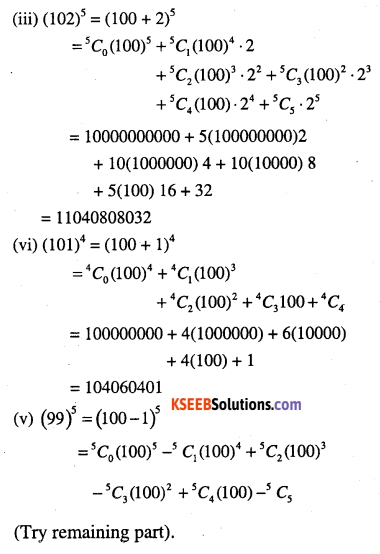Question 4.
Which is longer $$(1.01)^{1000000} \text { or } 10,000 ?$$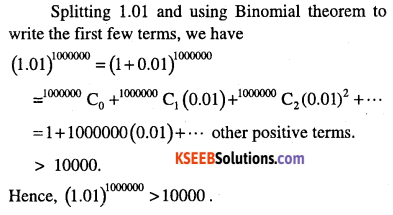Question 5.
Using Binomial theorem, indicate which number is larger $$(1.1)^{10000} \text { or } 1000 ?$$
Spilitting 1.01 and using Binomial theorem write the first few terms, we have.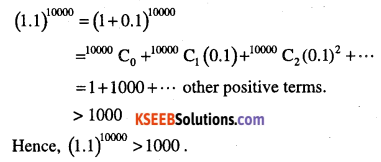Question 6.
Find (a + b)4 – (a -b)4. Hence evaluate
$$(\sqrt{3}+\sqrt{2})^{4}-(\sqrt{3}-\sqrt{2})^{4}$$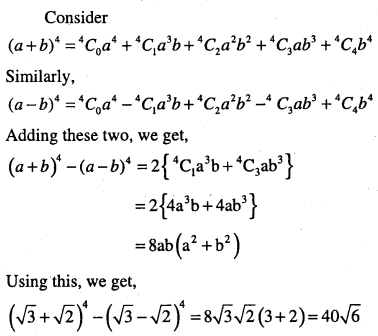Question 7.
Find (x +1)6 +(x -1)6. Hence or otherwise evaluate
$$(\sqrt{2}+1)^{6}+(\sqrt{2}-1)^{6}$$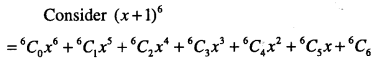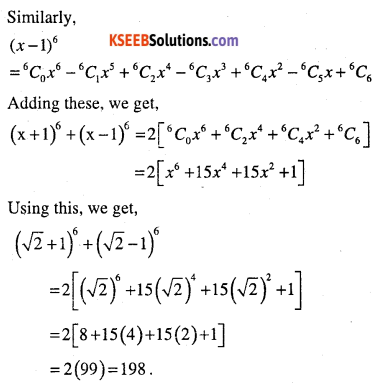Question 8.
Evaluate:
$$(\sqrt{3}+\sqrt{2})^{6}+(\sqrt{3}-\sqrt{2})^{6}$$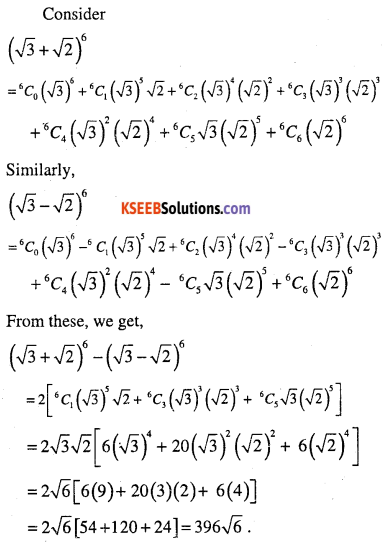Question 9.
Find the value of
$$\left(a^{2}+\sqrt{a^{2}-1}\right)^{4}+\left(a^{2}-\sqrt{a^{2}-1}\right)^{4}$$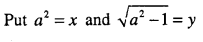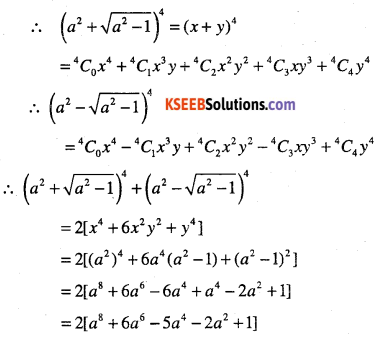Question 10.
Show that 9n+1 – 8n – 9 is divisible by 64, whenever ‘n’ is a positive integer.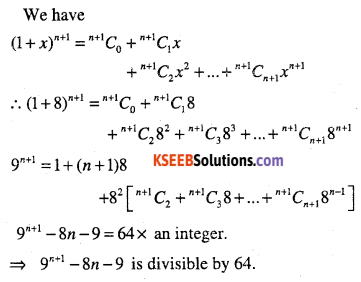Question 11.
Using binomial theorem, prove that 6n -5n always leaves remainder 1 when
divided by 25.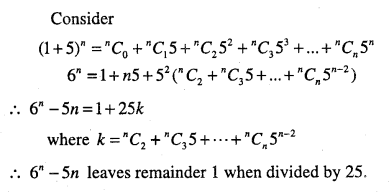Question 12.
Prove that
$$\sum_{n=0}^{n} y \cdot c_{n}=4$$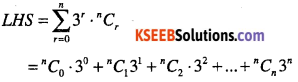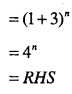Question 13.
Find the 4th term in the expansion of (x-2 y)12.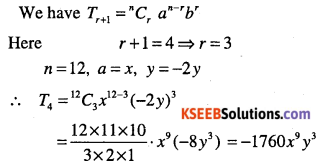Question 14.
Find the 13th term in the expansion of
$$\left(9 x-\frac{1}{3 \sqrt{x}}\right)^{18}, x \neq 0$$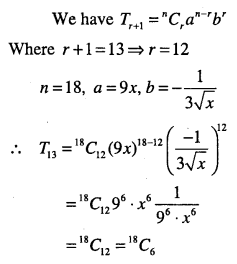Question 15.
Write the general term in the expression of
(i) (x2 -y)6
(ii) (x2-yx)12,x≠0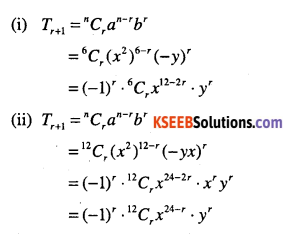Question 16.
Find the coefficient of
(i) x5 in (x + 3)8
(ii) a5b7 in (a – 2b)12
(iii) x6y3 in (x + 2y)9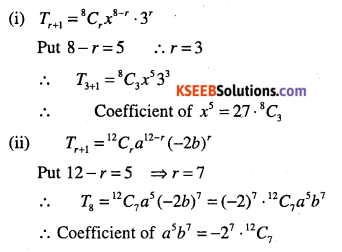Question 17.
Find a, if the 17th and 18th terms of the expansion (2 +a)50 are equal.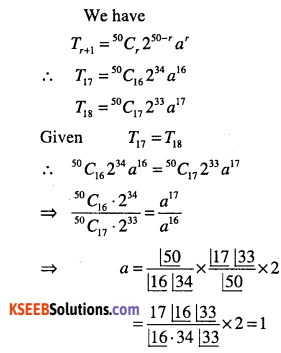Question 18.
In the expansion of (1+ a)m+n, prove that coefficients of am and an are equal.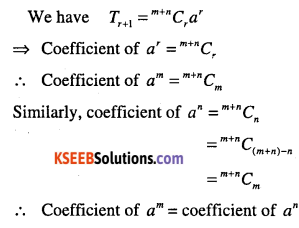Question 19.
Prove that the coefficient of xn in the expansion of (1 + x)2n is twice the coefficient of xn in the expansion of (1+x)2n-1
In (1+x)2n we have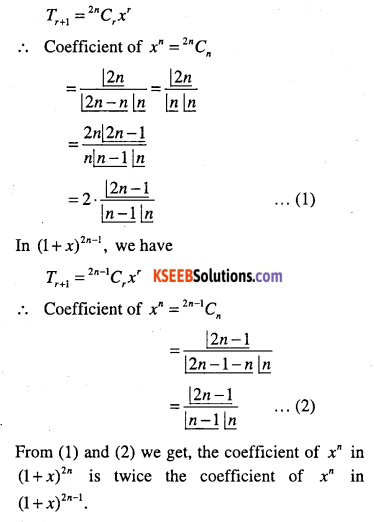From (1) and (2) we get, the coefficient of xn in (1 + x)2n is twice the coefficient of xn in (1 + x)2n-1.Question 20.
Find a positive value of m for which the coefficient of x2 in the expansion (1 + x)m is 6.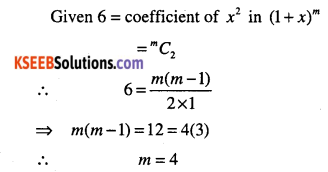Question 21.
Find the middle terms in the expansions of
(i) $$\left(3-\frac{x^{3}}{6}\right)^{7}$$
(ii)$$\left(\frac{x}{3}+9 y\right)^{10}$$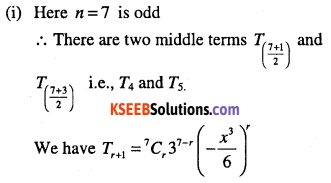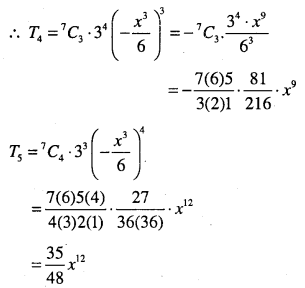Question 22.
Show that the middle term in the expansion of $$(1+x)^{2 n} \text { is } \frac{1 \cdot 3 \cdot 5 \ldots(2 n-1)}{\lfloor n} 2^{n} x^{n}$$where ‘n’ is a positive integer.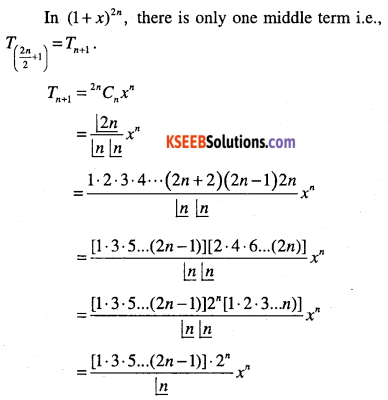Question 23.
The second, third and fourth terms in the binomial expansion (x + a)n are 240, 720 and 1080, respectively. Find x, a and n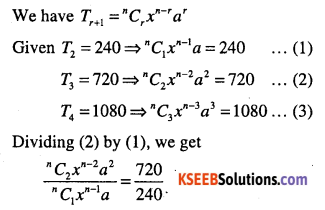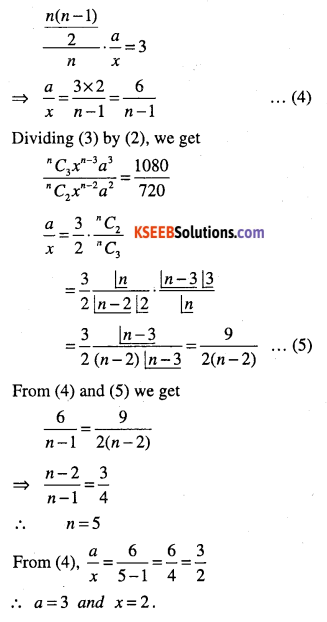Question 24.
The coefficients of three consecutive terms in the expansion of (1 + a)n are in the ratio 1:7: 42. Find n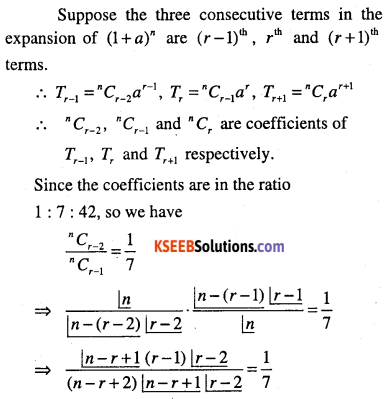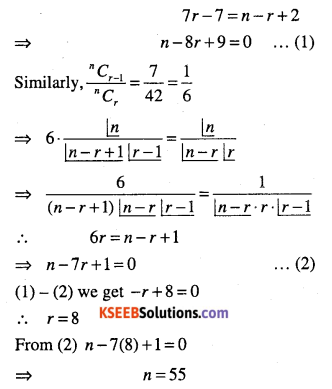Question 25.
The coefficients (r-1)th, rth,and (r + 1)th, terms in the expansion of (x + 1)th, are in the ratio 1:3:5. Find n and r.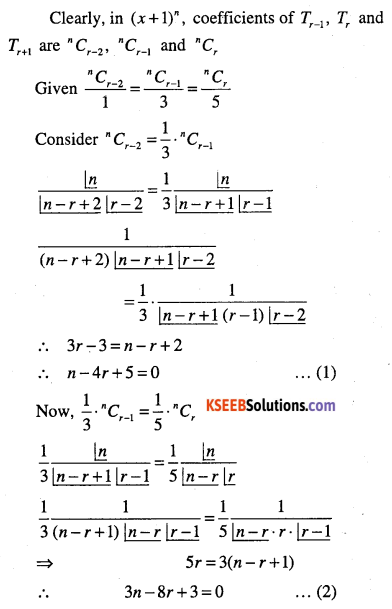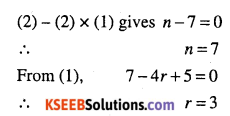Question 26.
Find the term independent of x in the expansion of $$\left(\frac{3}{2} x^{2}-\frac{1}{3 x}\right)^{6}$$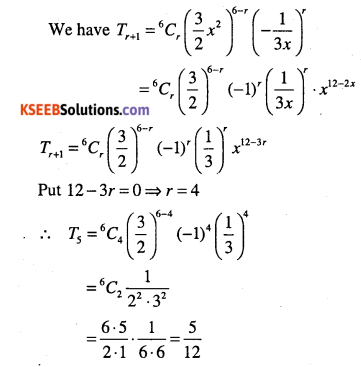Question 27.
Find the term independent of x in the expansion of $$\left(\sqrt{x}+\frac{1}{2 \sqrt{x}}\right)^{18}, x>0$$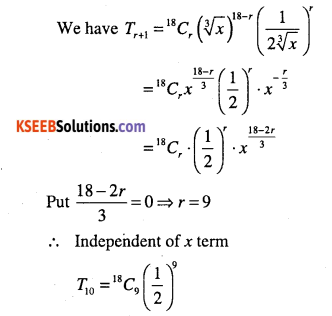Question 28.
Find a, 6 and n in the expansion of (a + b)n if the first three terms of the expansion are 729,7290 and 30375, respectively.
Given: Tx = 729, T2 = 7290 and T3 = 30375
∴ an=729……………….(1)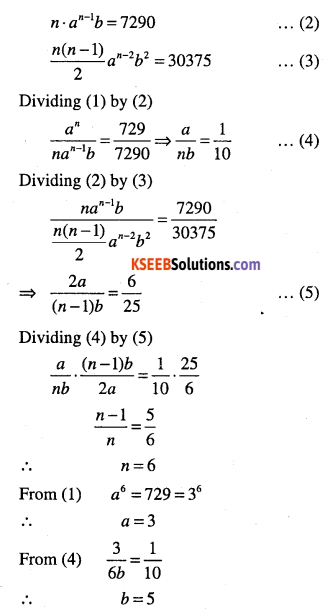Question 29.
Find a if the coefficients of x2 and x3 in the expansion of (3 +ax)9 are equal.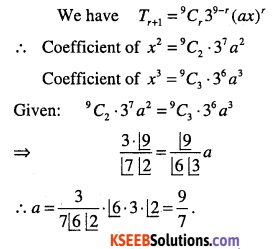Question 30.
If the coefficients of (r – 5)th and (2r -1)th terms in the expansion of (1 + x)34 are equal, find r.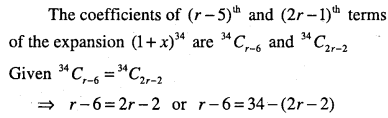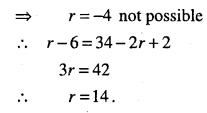Question 31.
If the coefficients of ar-1, ar and ar+1 in the expansion of (1 + a)n are in arithmetic progression, prove that n2 – n(4r +1) + 4r2 -2 = 0.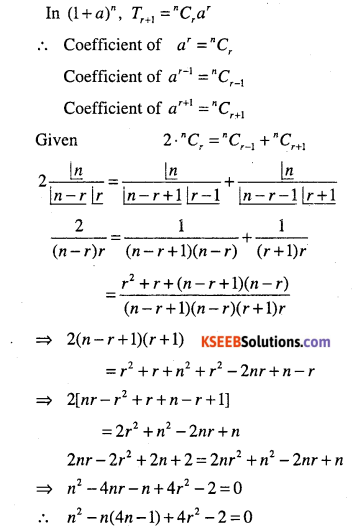Question 32.
Show that the coefficient of the middle term in the expansion of (1 + x)2n is equal to the sum of the coefficients of two middle terms in the expansion of (1 + x)2n-1.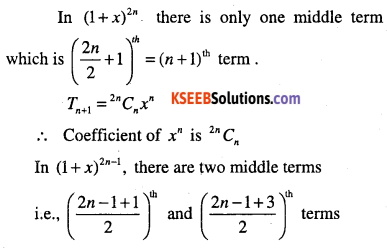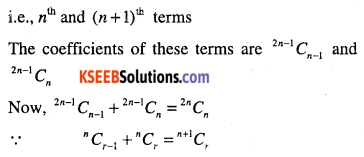Question 33.
Find n, if the ratio of the fifth term from the beginning to the fifth term from the end in the expansion of
$$\left(\sqrt{2}+\frac{1}{\sqrt{3}}\right)^{n} \text { is } \sqrt{6}: 1$$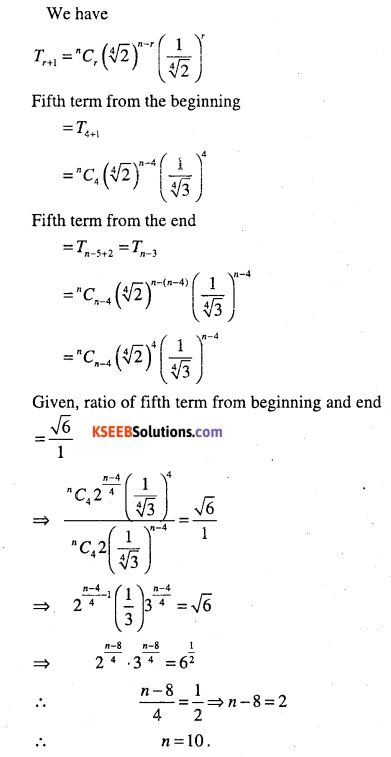Question 34.
Find the rth term from the end in the expansion of (x + a)n.
rth term from the end in (x + a)n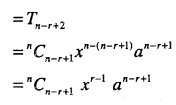Question 35.
If a and b are distinct integers, prove that a-b is a factor of an -bn, whenever n is a positive integer.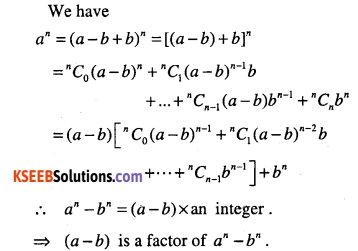Question 36.
The sum of the coefficients of the first three terms in the expansion of
$$\left(x-\frac{3}{x^{2}}\right)^{m}, x \neq 0, m$$being a natural numbers, is 559. Find the term of the expansion containing x3.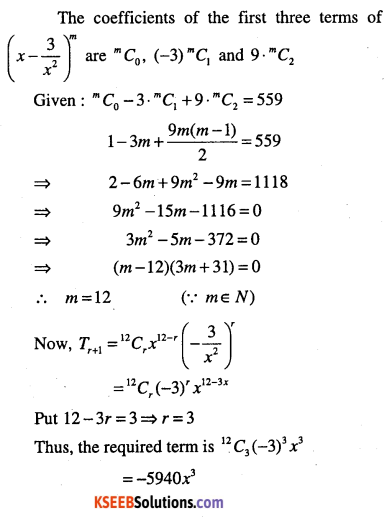Question 37.
Find the coefficient of x5 in the product (1 + 2x)6(1 – x)7 using binomial theorem.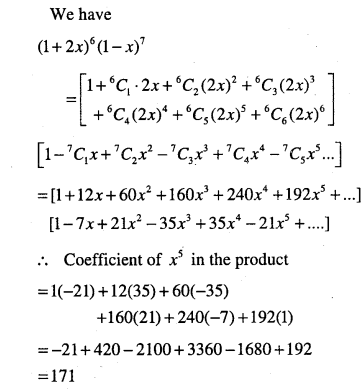Question 38.
Find the coefficient of a4 in the product (1 + 2a)4(2-a)s using binomial theorem.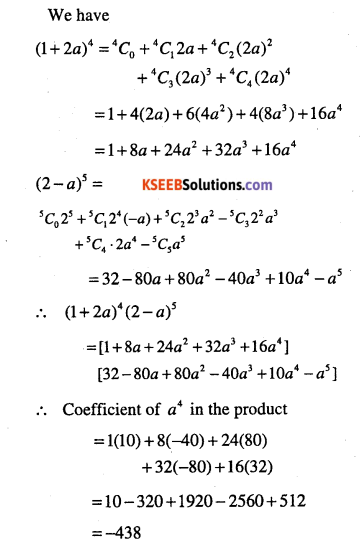Question 39.
Expand using Binomial theorem
$$\left(1+\frac{x}{2}-\frac{2}{x}\right)^{4}, x \neq 0$$